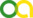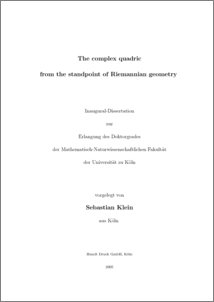Klein, Sebastian (2005). The complex quadric from the standpoint of Riemannian geometry. PhD thesis, Universität zu Köln.Preview
PDF
Diss.pdf

Download (1MB)

## Abstract

The complex hypersurfaces of a complex projective space P^n which are of least complexity (apart from the projective subspaces, whose geometry is known completely) are those which are determined by a non-degenerate quadratic equation, the complex quadrics. From the algebraic point of view, all complex quadrics are equivalent. However, if one regards P^n as a Riemannian manifold (with the Fubini-Study metric), it turns out that only certain complex quadrics are adapted to the Riemannian metric of P^n in the sense that they are symmetric submanifolds of P^n. These quadrics are also singled out by the fact that they are (again apart from the projective subspaces) the only complex hypersurfaces in P^n which are Einstein manifolds, see see Smyth, [Smy67] (the bibliographic references of the present abstract refers to the bibliography of the dissertation). In the sequel the term ``complex quadric'' always refers to these adapted complex quadrics. While the algebraic behaviour of complex quadrics Q is well-known, there still remains a lot to be said about their intrinsic and extrinsic Riemannian geometry; the present dissertation provides a contribution to this subject. Specifically, the following results are obtained: -- The classification of the totally geodesic submanifolds of Q. -- The investigation of certain congruence families of totally geodesic submanifolds in Q; these families are equipped with the structure of a naturally reductive homogeneous space in a general setting, and it is investigated in which cases this structure is induced by a symmetric structure. -- It is shown that the set of the k-dimensional ``subquadrics'' contained in Q (which are all isometric to one another) is composed of a one-parameter-series of congruence classes; moreover the extrinsic geometry of these subquadrics is studied. -- It is well known that the following isomorphies hold between complex quadrics of low dimension and members of other series of Riemannian symmetric spaces: Q^1 is isomorphic to the sphere S^2 Q^2 is isomorphic to P^1 x P^1 Q^3 is isomorphic to Sp(2)/U(2) Q^4 is isomorphic to the Grassmannian G_2(C^4) Q^6 is isomorphic to SO(8)/U(4) These isomorphisms are constructed explicitly in a rather geometric way.

Item Type: Thesis (PhD thesis)
Translated title:
TitleLanguage
Die komplexe Quadrik vom Standpunkt der Riemannschen GeometrieGerman
Creators:
CreatorsEmailORCID
Klein, Sebastiansklein@math.uni-koeln.deUNSPECIFIED
URN: urn:nbn:de:hbz:38-16273
Subjects: Mathematics
Uncontrolled Keywords:
KeywordsLanguage
symmetrische Räume , totalgeodätische Untermannigfaltigkeiten , komplexe Quadrik , Riemannsche GeometrieGerman
symmetric spaces , totally geodesic submanifolds , complex quadric , Riemannian geometryEnglish
Faculty: Faculty of Mathematics and Natural Sciences
Divisions: Faculty of Mathematics and Natural Sciences > Mathematical Institute
Language: English
Date: 2005
Date of oral exam: 31 January 2005
Referee:
NameAcademic Title
Reckziegel, HelmutProf. Dr.
Refereed: Yes
URI: http://kups.ub.uni-koeln.de/id/eprint/1627

### Downloads

Downloads per month over past year

### Actions (login required)View Item